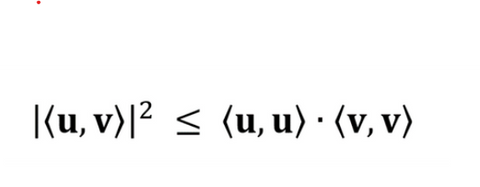If u and v are vectors defined over an inner product space (a vector space combined with a structure called an ‘inner product’ that satisfies certain conditions), then the above Cauchy-Schwarz inequality is true.

This is a general statement for a very widely used tool in mathematics. It might be easier to visualise in the context of the inner product space of the real numbers in the nth dimension, where the inner product is defined as the more commonly known ‘dot product’. There are several equations in varying areas of mathematics that follow as a direct consequence of Cauchy-Schwarz, such as the triangle inequality in analysis, which determines the angle between two vectors in geometry and the covariance inequality in probability theory.

The inequality for sums was first published by Augustin-Louis Cauchy in 1821 and later for integrals by Hermann Schwarz in 1888, hence ‘Cauchy-Schwarz’. Augustin-Louis Cauchy was a French mathematician, engineer, and physicist. A prolific writer, he wrote approximately 800 research articles and five complete textbooks on a variety of topics and consequently his name appears before more theorems and concepts than perhaps any other mathematician. The lesser-known German mathematician Schwarz originally studied chemistry; however, he was convinced to change to mathematics by his father-in-law, the famous mathematician Ernst Eduard Kummer.